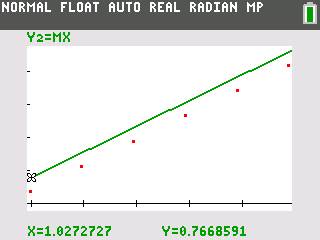### Step by Step

Students take distance readings as they walk in front of a CBR™. They apply the properties of a linear function to develop a model for the motion and interpret the values used in the model. Students learn to graph scatter plots, calculate slope, and graph linear function.
•TI-84 Plus CE
•TI-84 Plus C Silver Edition
• TI-84 Plus Silver Edition
• TI-84 Plus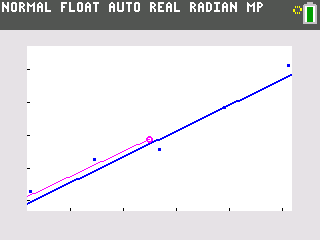Students explore the total voltage provided by several batteries in a series to a battery-operated device. They graph scatter plots, understand multiplication as repeated addition, and use a pattern to develop a formula. They learn how to develop a formula from a pattern of increasing voltage and graph increase in voltage in the form of a scatter plot.
•TI-84 Plus CE
•TI-84 Plus C Silver Edition
• TI-84 Plus Silver Edition
• TI-84 Plus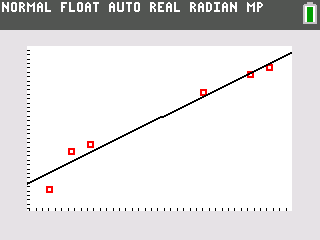### Steppin' Out

Students will use the Data/Graphs Wizard tool to graph data and determine a line that best fits your data and use the Data/Graphs Wizard tool to identify the relationship between stride length and height.
•TI-84 Plus CE
•TI-84 Plus C Silver Edition
• TI-84 Plus Silver Edition
• TI-84 PlusStudents will model data using a linear equation, interpret the slope and intercept values from a linear model, and identify a characteristic property of a substance.
•TI-84 Plus CE
•TI-84 Plus C Silver Edition
• TI-84 Plus Silver Edition
• TI-84 Plus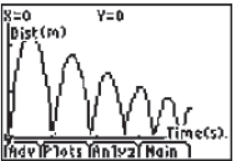### Bouncing Ball

In this experiment, students collect the height versus time data of a bouncing ball using the CBR 2™. This activity investigates the values of height, time, and the coefficient A in the quadratic equation, Y = A(X - H)2 + K, which describes the behavior of a bouncing ball. They graph scatter plots, graph and interpret a quadratic function, apply the vertex form of a quadratic equation, and calculate the maximum value of a parabola.
•TI-84 Plus CE
•TI-84 Plus C Silver Edition
• TI-84 Plus Silver Edition
• TI-84 Plus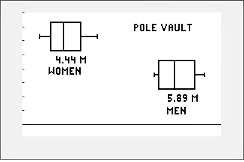### Science TODAY™ - Clearing the Bar

Students will explore the effects of two critical physical factors on potential success in the pole vault event in track and field.
• TI-84 Plus Silver Edition
• TI-84 Plus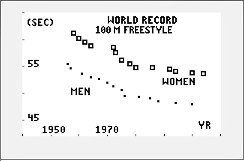### Science TODAY™ - How the Olympic Swimsuit Gives Athletes an Edge

Students will look at the potential impact of an improvement in the equipment used by world-class swimmers. They will also look at the improvement in the world records for men and women in the 100 Meter Freestyle race.
• TI-84 Plus Silver Edition
• TI-84 Plus### Science TODAY™ - Hurricane Damage

Students will construct a graph and a regression model which shows the relationship between wind speed and the force of the wind. The students will then analyze the math model that fits the data to make predictions from the model.
• TI-84 Plus Silver Edition
• TI-84 Plus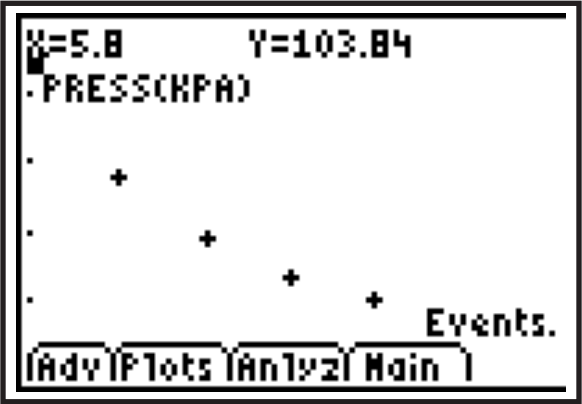### Can You Take The Pressure?

Students will use the Vernier EasyLink© and the Gas Pressure Sensor to determine the volume of a gas in a container under different pressures. They will also graph the pressure/volume data and interpret their graphs.
•TI-84 Plus CE
•TI-84 Plus C Silver Edition
• TI-84 Plus Silver Edition
• TI-84 Plus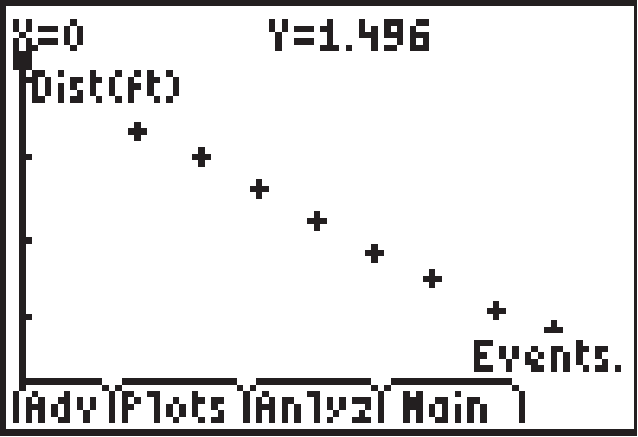### Hooke's Law

Students study Hooke's Law. They understand that stretch on a spring is directly proportional to the force applied to the end of the string. Students observe a spring being stretched as candies are added to a cup attached to the spring. They learn to graph scatter plots, analyze linear function, calculate and model slope.
•TI-84 Plus CE
•TI-84 Plus C Silver Edition
• TI-84 Plus Silver Edition
• TI-84 Plus### Hooke's Law: The Rest of the Story

Students study Hooke's Law. They explore the linear behavior of a spring as it is pulled downward and understand that stretch on a spring is directly proportional to the force applied to the end of the string. Students create scatter plots, calculate slopes, and discuss positive and negative slopes.
•TI-84 Plus CE
•TI-84 Plus C Silver Edition
• TI-84 Plus Silver Edition
• TI-84 Plus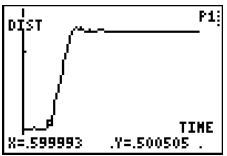### Vernier - Air Resistance

Students use the Vernier Motion Detector to measure the effect of air resistance on falling objects. They determine how air resistance and mass affect the terminal velocity of a falling object and then choose a force model that fits the data.
•TI-84 Plus CE
•TI-84 Plus C Silver Edition
• TI-84 Plus Silver Edition
• TI-84 Plus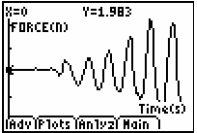### Vernier - An Inclined Plane

In this activity, students will use a Force Sensor to measure the force needed to lift an object and the force needed to pull the same object up an inclined plane. They will also calculate work done and efficiency and make conclusions.
•TI-84 Plus CE
•TI-84 Plus C Silver Edition
• TI-84 Plus Silver Edition
• TI-84 Plus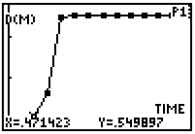### Vernier - Falling Objects

In this activity, students will use a Motion Detector to measure distance and velocity.
•TI-84 Plus CE
•TI-84 Plus C Silver Edition
• TI-84 Plus Silver Edition
• TI-84 Plus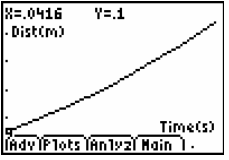### Vernier - Picket Fence Free Fall

In this activity, students will measure the acceleration of a freely falling body (g) to better than 0.5% precision with the help of a Picket Fence and a Photogate.
•TI-84 Plus CE
•TI-84 Plus C Silver Edition
• TI-84 Plus Silver Edition
• TI-84 Plus### Vernier - The Magnetic Field in a Slinky

Students use a Magnetic Field Sensor to measure the magnetic field and the current in a solenoid. They explore factors that affect the magnetic field, study how the field varies in parts of the solenoid, and determine the permeability constant.
•TI-84 Plus CE
•TI-84 Plus C Silver Edition
• TI-84 Plus Silver Edition
• TI-84 Plus### Vernier - Bungee Jump Accelerations

Students use an Accelerometer to analyze the motion of a bungee jumper and determine a point where there is maximum and minimum acceleration in the course of the motion. They also compare the experimental data with an actual bungee jump.
•TI-84 Plus CE
•TI-84 Plus C Silver Edition
• TI-84 Plus Silver Edition
• TI-84 Plus